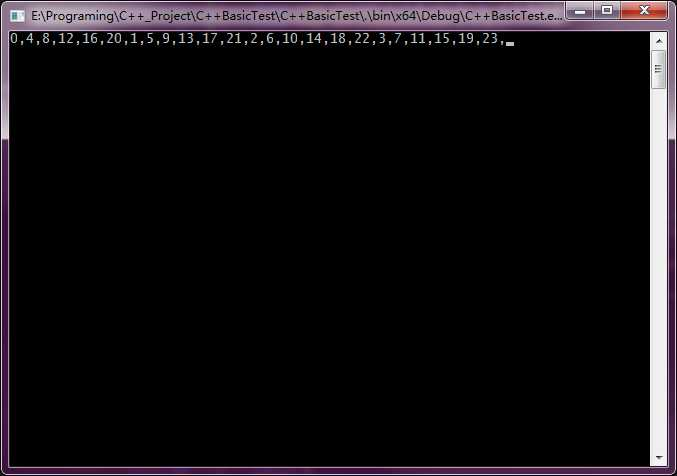# 1 C++实现Python numpy的矩阵维度转置算法，例如(N,H,W,C)转换为(N,C,H,W)

## 1.1 python中使用numpy进行矩阵维度变换

import numpy as np

if __name__ == '__main__':
a = np.arange(0,24,1)

N = 1
H = 2
W = 3
C = 4

a = np.reshape(a,[1,2,3,4])
print(a)

a_copy = a
test_copy = a

print(a.flatten())

# N.H,W,C -> N,C,H,W
a_copy = np.transpose(a_copy,[0,3,1,2])

print(a_copy.flatten())

# 使用数组transpose
a_new = []
for n in range(N):
for c in range(C):
for h in range(H):
for w in range(W):
a_new.append(test_copy[n,h,w,c])

print(a_new)


[[[[ 0  1  2  3]
[ 4  5  6  7]
[ 8  9 10 11]]

[[12 13 14 15]
[16 17 18 19]
[20 21 22 23]]]]
[ 0  1  2  3  4  5  6  7  8  9 10 11 12 13 14 15 16 17 18 19 20 21 22 23]
[ 0  4  8 12 16 20  1  5  9 13 17 21  2  6 10 14 18 22  3  7 11 15 19 23]
[0, 4, 8, 12, 16, 20, 1, 5, 9, 13, 17, 21, 2, 6, 10, 14, 18, 22, 3, 7, 11, 15, 19, 23]


[[[[ 0  1  2  3]
[ 4  5  6  7]
[ 8  9 10 11]]

[[12 13 14 15]
[16 17 18 19]
[20 21 22 23]]]]


，事实上该四维矩阵在内存中的排列如下：

[ 0  1  2  3  4  5  6  7  8  9 10 11 12 13 14 15 16 17 18 19 20 21 22 23]


[ 0  4  8 12 16 20  1  5  9 13 17 21  2  6 10 14 18 22  3  7 11 15 19 23]


## 1.2 在C++中对矩阵进行维度变换

#include <iostream>
#include <string>

int main()
{
int N = 1;
int H = 2;
int W = 3;
int C = 4;

float temp_int = { 0, 1, 2, 3, 4,5,6,7,8,9,10,11,12,13,14,15,16,17,18,19,20,21,22,23 };
float temp_int_transpose = { 0 };

// [N,H,W,C] -> [N,C,H,W]

for (int n = 0; n < N; ++n)
{
for (int c = 0; c < C; ++c)
{
for (int h = 0; h < H; ++h)
{
for (int w = 0; w < W; ++w)
{
int old_index = n * H * W * C + h * W * C + w * C + c;
int new_index = n * C * H * W + c * H * W + h * W + w;

temp_int_transpose[new_index] = temp_int[old_index];

std::cout << temp_int_transpose[new_index] << ",";
}
}
}
}

getchar();

return 0;
}